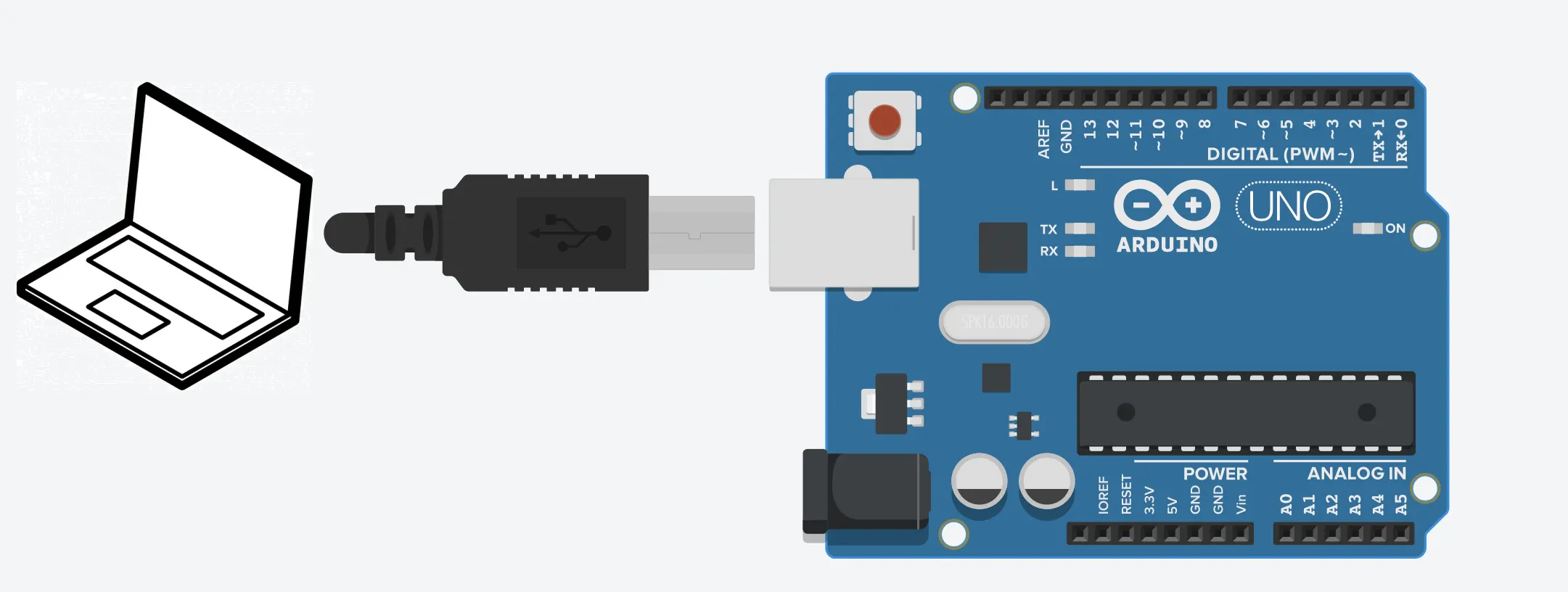Run a Virtual Factory with Arduino!

This is an excellent way to learn about Arduino Without hardware. The only thing you need is an Arduino Mega, Uno or Nano.

IntermediateFull instructions provided1 hour1,566

Things used in this project

Hardware componentsArduino UNO
×1

Software apps and online servicesArduino IDE

Schematics

Arduino connected with a USB cable to PCCode

Software_converter_Modbus

Python
This is an executable file made of a Python script that will parse the data of a Modbus serial client to a Modbus TCP/IP server.

Not_Needed_Source_Code_Modbus_converter

Python
This is the Python scrip used for the exe. file to parse Modbus serial data to Modbus TCP/IP.
I have no formal training in text based coding and I'm used to PLC language based coding so please excuse me for the bad syntax and stuff. I'm just glad that it worked (put some time and effort in it).
#Script to read a serial Modbus Client Arduino and parse the data to TCP/IP Modbus
#client to Factory I/O.
#Author : Seafoxc Date: 15/05/2021
#Please note that I'm not an IT person by education and that I know the script can be optimised a lot.
#But it works for the intended purpose so that is all it has to do for me.

from pymodbus.client.sync import ModbusSerialClient as ModbusClient
from pymodbus.client.sync import ModbusTcpClient
import time
from tkinter import *

from argparse import ArgumentParser
from socket import create_connection
from umodbus import conf
from umodbus.client import tcp

# Enable values to be signed (default is False).
conf.SIGNED_VALUES = True

master = Tk()
text = Text(master, height=8, width=46)
text.insert(INSERT, "Arduino to Factory I/O Modbus connector.\n \nAfter choosing the connection parameters closethe window.\n\nSeafoxC 2021")

text.pack()

COM = StringVar(master)
COM.set("COM1") # default value

w = OptionMenu(master, COM, "COM1", "COM2", "COM3","COM4", "COM5", "COM6", "COM7", "COM8", "COM9", "COM10")
w.pack()

IP = StringVar(master)
IP.set("192.168.0.132") # default value

w = OptionMenu(master, IP, "192.168.0.132", "192.168.0.133", "192.168.0.134","192.168.0.135", "192.168.0.136", "192.168.0.137", "192.168.0.138", "192.168.0.139", "192.168.0.140", "192.168.0.141")
w.pack()

mainloop()

COM  = COM.get()
print(COM)

IP  = IP.get()
print(IP)

port = int(502)

#Set-up for the Serial Modbus client (the Arduino).
client = ModbusClient(method='rtu', port= COM ,  baudrate=115200, timeout = 100, parity = 'N', stopbits=1,  bytesize=8)
connection = client.connect()
if connection == True:
print("Connected to "+ COM )
else:
print("Not connected to Arduino check COM port and if firmware is loaded on device")

time.sleep(2)

port = int(502)

FactoryIO_Input0_prev = False
FactoryIO_Input1_prev = False
FactoryIO_Input2_prev = False
FactoryIO_Input3_prev = False
FactoryIO_Input4_prev = False
FactoryIO_Input5_prev = False
FactoryIO_Input6_prev = False
FactoryIO_Input7_prev = False
FactoryIO_Input8_prev = False
FactoryIO_Input9_prev = False
FactoryIO_Input10_prev = False
FactoryIO_Input11_prev = False
FactoryIO_Input12_prev = False
FactoryIO_Input13_prev = False
FactoryIO_Input14_prev = False
FactoryIO_Input15_prev = False

while True:

#Read Factory I/O Inputs from Modbus TCP/IP client.
# Returns a message or Application Data Unit (ADU) specific for doing
# Modbus TCP/IP.

with create_connection((IP, 502)) as sock:

#Write Factory Inputs to Coils 0-16 to Arduino.
#Only write a coil when the input is different from the previous run (otherwise very slow).

if FactoryIO_Input0 != FactoryIO_Input0_prev:
client.write_coil(0,FactoryIO_Input0)
FactoryIO_Input0_prev = FactoryIO_Input0

if FactoryIO_Input1 != FactoryIO_Input1_prev:
client.write_coil(1,FactoryIO_Input1)
FactoryIO_Input1_prev = FactoryIO_Input1

if FactoryIO_Input2 != FactoryIO_Input2_prev:
client.write_coil(2,FactoryIO_Input2)
FactoryIO_Input2_prev = FactoryIO_Input2

if FactoryIO_Input3 != FactoryIO_Input3_prev:
client.write_coil(3,FactoryIO_Input3)
FactoryIO_Input3_prev = FactoryIO_Input3

if FactoryIO_Input4 != FactoryIO_Input4_prev:
client.write_coil(4,FactoryIO_Input4)
FactoryIO_Input4_prev = FactoryIO_Input4

if FactoryIO_Input5 != FactoryIO_Input5_prev:
client.write_coil(5,FactoryIO_Input5)
FactoryIO_Input5_prev = FactoryIO_Input5

if FactoryIO_Input6 != FactoryIO_Input6_prev:
client.write_coil(6,FactoryIO_Input6)
FactoryIO_Input6_prev = FactoryIO_Input6

if FactoryIO_Input7 != FactoryIO_Input7_prev:
client.write_coil(7,FactoryIO_Input7)
FactoryIO_Input7_prev = FactoryIO_Input7

if FactoryIO_Input8 != FactoryIO_Input8_prev:
client.write_coil(8,FactoryIO_Input8)
FactoryIO_Input8_prev = FactoryIO_Input8

if FactoryIO_Input9 != FactoryIO_Input9_prev:
client.write_coil(9,FactoryIO_Input9)
FactoryIO_Input9_prev = FactoryIO_Input9

if FactoryIO_Input10 != FactoryIO_Input10_prev:
client.write_coil(10,FactoryIO_Input10)
FactoryIO_Input10_prev = FactoryIO_Input10

if FactoryIO_Input11 != FactoryIO_Input11_prev:
client.write_coil(11,FactoryIO_Input11)
FactoryIO_Input11_prev = FactoryIO_Input11

if FactoryIO_Input12 != FactoryIO_Input12_prev:
client.write_coil(12,FactoryIO_Input12)
FactoryIO_Input12_prev = FactoryIO_Input12

if FactoryIO_Input13 != FactoryIO_Input13_prev:
client.write_coil(13,FactoryIO_Input13)
FactoryIO_Input13_prev = FactoryIO_Input13

if FactoryIO_Input14 != FactoryIO_Input14_prev:
client.write_coil(14,FactoryIO_Input14)
FactoryIO_Input14_prev = FactoryIO_Input14

if FactoryIO_Input15 != FactoryIO_Input15_prev:
client.write_coil(15,FactoryIO_Input15)
FactoryIO_Input15_prev = FactoryIO_Input15

#Read Factory Input register from Modbus TCP/IP client.
# Returns a message or Application Data Unit (ADU) specific for doing
# Modbus TCP/IP.

with create_connection(('192.168.0.132', 502)) as sock:

# Read the Coils from Arduino. The coils from 16 to 32 are for writing the outputs.
#print("This is coil 2 " ,Coils.bits)

#Extract the bits from the coils.
FactoryIO_Output0 = Coils.bits
FactoryIO_Output1 = Coils.bits
FactoryIO_Output2 = Coils.bits
FactoryIO_Output3 = Coils.bits
FactoryIO_Output4 = Coils.bits
FactoryIO_Output5 = Coils.bits
FactoryIO_Output6 = Coils.bits
FactoryIO_Output7 = Coils.bits
FactoryIO_Output8 = Coils.bits
FactoryIO_Output9 = Coils.bits
FactoryIO_Output10 = Coils.bits
FactoryIO_Output11 = Coils.bits
FactoryIO_Output12 = Coils.bits
FactoryIO_Output13 = Coils.bits
FactoryIO_Output14 = Coils.bits
FactoryIO_Output15 = Coils.bits

FactoryIO_Outputs_list = [FactoryIO_Output0, FactoryIO_Output1,FactoryIO_Output2,FactoryIO_Output3,FactoryIO_Output4,FactoryIO_Output5,FactoryIO_Output6,FactoryIO_Output7,
FactoryIO_Output8,FactoryIO_Output9,FactoryIO_Output10,FactoryIO_Output11,FactoryIO_Output12,FactoryIO_Output13,FactoryIO_Output14,FactoryIO_Output15]

# Read the Holding register from Arduino.
#the list with the values is rr.register

#Code for Modbus TCP-IP client
# Returns a message or Application Data Unit (ADU) specific for doing
# Modbus TCP/IP.
message2 = tcp.write_multiple_registers(slave_id=2, starting_address=0, values= (rr.registers))
with create_connection(('192.168.0.132', 502)) as sock:
response1 = tcp.send_message(message1, sock)
response1 = tcp.send_message(message2, sock)

Arduino_FactoryIO_Empty.zip

C/C++
ZIP file. Make sure to extract it!

C/C++
This is a ZIP file. Make sure to Unzip it!